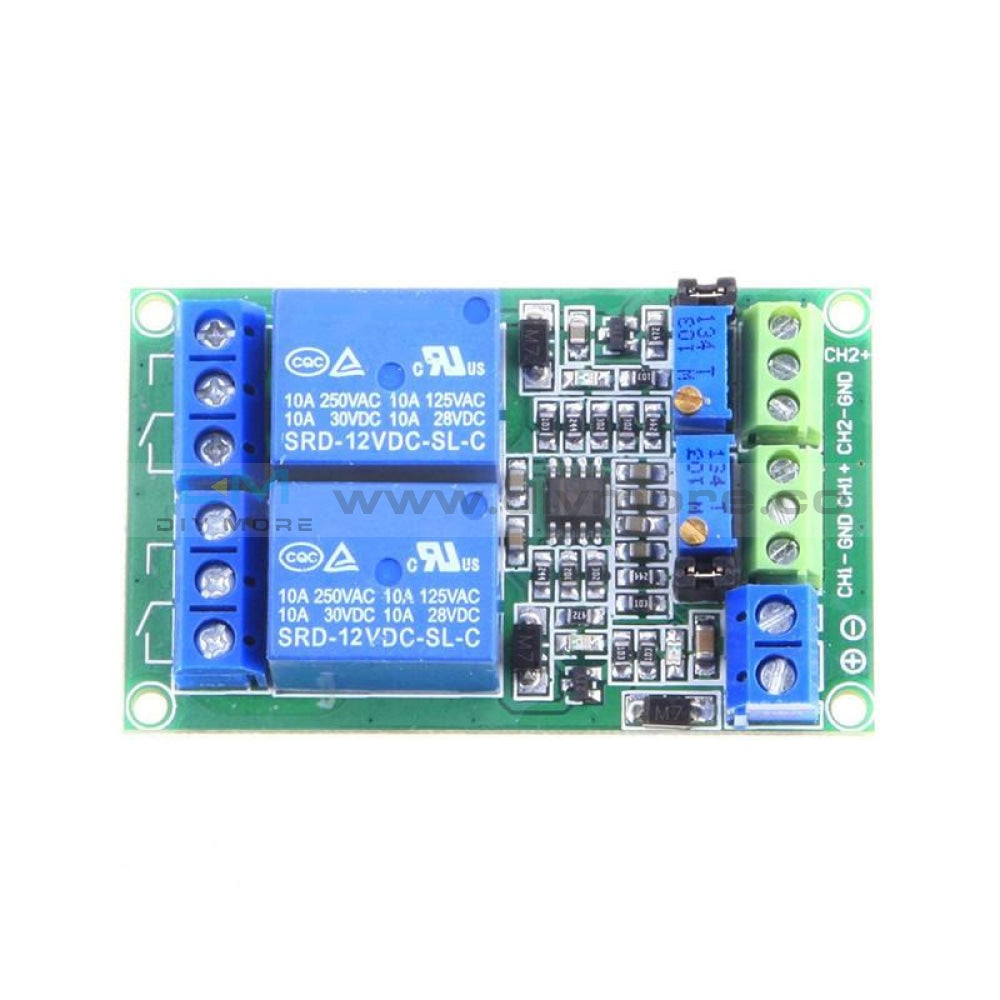### DC 12V 2-Channel Voltage Comparator Precise LM393 Module### DC 12V 2-Channel Voltage Comparator Precise LM393 Module

SKU:011264
Regular price \$6.99Specification:
Working voltage: DC12V.
Working current: DC12V 52mA
Size:63*38mm

Function:
The setting voltage reference input of a voltage and on the module (reference voltage can be set through the adjustable resistor) the contrast,
when the input voltage is greater than the reference voltage, the relay is closed (11-10 closed, 11-12 disconnected), when the input voltage is less than the reference voltage, relay does not operate.
To compare respectively input voltage signals A and B, when the voltage signal A is greater than the voltage signal B, the relay is closed (11-10 closed, 11-12 disconnected), when the voltage signal A is less than B voltage signal
The setting voltage reference input of a voltage and on the module (reference voltage can be set through the adjustable resistor) the contrast, when the input voltage is less than the reference voltage,
the relay is closed (11-10 closed, 11-12 disconnected), when the input voltage is greater than the reference voltage, after the electric appliance does not work.

The Reference Voltage:
Voltage reference module (1 or 2-3 input voltage value) after precision resistor divider, input voltage value determines the range of the reference voltage value,
Such as the input voltage is 12V, then the reference voltage adjustable range is 0-12V

Module Purpose:
The automobile circuit hardware, industrial equipment, laboratory testing, application testing circuit.

Package Included:
1 x  2-Channel Voltage Comparator Module• guaranteeQuality checked
• Free return Within 60 days
• Consultancy86-0755-85201155
Specification:
Working voltage: DC12V.
Working current: DC12V 52mA
Size:63*38mm

Function:
The setting voltage reference input of a voltage and on the module (reference voltage can be set through the adjustable resistor) the contrast,
when the input voltage is greater than the reference voltage, the relay is closed (11-10 closed, 11-12 disconnected), when the input voltage is less than the reference voltage, relay does not operate.
To compare respectively input voltage signals A and B, when the voltage signal A is greater than the voltage signal B, the relay is closed (11-10 closed, 11-12 disconnected), when the voltage signal A is less than B voltage signal
The setting voltage reference input of a voltage and on the module (reference voltage can be set through the adjustable resistor) the contrast, when the input voltage is less than the reference voltage,
the relay is closed (11-10 closed, 11-12 disconnected), when the input voltage is greater than the reference voltage, after the electric appliance does not work.

The Reference Voltage:
Voltage reference module (1 or 2-3 input voltage value) after precision resistor divider, input voltage value determines the range of the reference voltage value,
Such as the input voltage is 12V, then the reference voltage adjustable range is 0-12V

Module Purpose:
The automobile circuit hardware, industrial equipment, laboratory testing, application testing circuit.

Package Included:
1 x  2-Channel Voltage Comparator Module